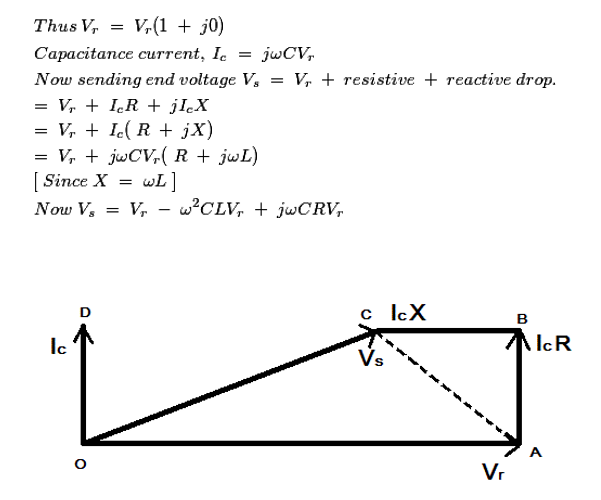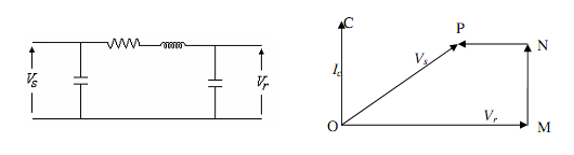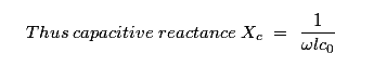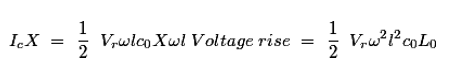Ferranti effect - Electrical Engineering Gate

# Electrical Engineering Gate

Online Electrical Engineering Study Site

## Friday, November 21, 2014

A long transmission line draws a substantial quantity of charging current. If such a line is open circuited or very lightly loaded at the receiving end the voltage at receiving end may become greater than voltage at sending end. This is known as Ferranti Effect and is due to the voltage drop across the line inductance being in phase with the sending end voltages. Therefore both capacitane and inductance is responsible to produce this phenomenon .
The capacitance and charging current is negligible in short line but significant in medium line and appreciable in in long line by equivalent π model
It is proportional to the square of lengths of lines, that is, ∆V α kx2
, where x is the length of line and k is a constant for all voltage levels
In general practice we know, that for all electrical systems electric current flows from the region of higher potential to the region of lower potential, to compensate for the electrical potential difference that exists in the system. In all practical cases the sending end voltage is higher than the receiving end, so electric current flows from the source or the supply end to the load. But Sir S.Z. Ferranti, in the year 1890, came up with an astonishing theory about medium distance transmission line or long distance transmission linessuggesting that in case of light loading or no load operation of transmission system, the receiving end voltage often increases beyond the sending end voltage, leading to a phenomena known as Ferranti effect in power system.

Why Ferranti Effect occurs in a Transmission Line?
long transmission line can be considered to composed a considerably high amount of capacitance and inductance distributed across the entire length of the line. Ferranti Effect occurs when current drawn by the distributed capacitance of the line itself is greater than the current associated with the load at the receiving end of the line( during light or no load). This capacitor charging current leads to voltage drop across the line inductance of the transmission system which is in phase with the sending end voltages. This voltage drop keeps on increasing additively as we move towards the load end of the line and subsequently the receiving end voltage tends to get larger than applied voltage leading to the phenomena called Ferranti effect in power system. It is illustrated with the help of a phasor diagram below.
Thus both the capacitance and inductance effect of transmission line are equally responsible for this particular phenomena to occur, and hence Ferranti effect is negligible in case of a short transmission lines as the inductance of such a line is practically considered to be nearing zero. In general for a 300 Km line operating at a frequency of 50 Hz, the no load receiving end voltage has been found to be 5% higher than the sending end voltage.
Now for analysis of Ferranti effect let us consider the phasor diagrame shown above.
Here Vr is considered to be the reference phasor, represented by OA.This is represented by the phasor OC.
Now in case of a , it has been practically observed that the line electrical resistance is negligibly small compared to the line reactance, hence we can assume the length of the phasor Ic R = 0, we can consider the rise in the voltage is only due to OA - OC = reactive drop in the line.Now if we consider c0 and L0 are the values of capacitance and inductance per km of the transmission line, where l is the length of the line.Since, in case of a , the capacitance is distributed throughout its length, the average current flowing is,Thus the rise in voltage due to line inductance is given by,From the above equation it is absolutely evident, that the rise in voltage at the receiving end is directly proportional to the square of the line length, and hence in case of a  it keeps increasing with length and even goes beyond the applied sending end voltage at times, leading to the phenomena called Ferranti effect in power system.